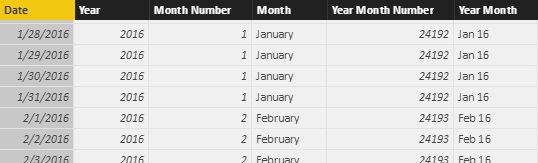A very popular DAX function to manipulate columns in a table expression is ADDCOLUMNS. You can use it to project new columns in a table expression. For example, this calculated table in Power BI generates a calendar table with columns for the year, month, and month number.

```Calendar 1 =
VAR Days = CALENDAR ( DATE ( 2016, 1, 1 ), DATE ( 2018, 12, 31 ) )
Days,
"Year", YEAR ( [Date] ),
"Month Number", MONTH ( [Date] ),
"Month", FORMAT ( [Date], "mmmm" ),
"Year Month Number", YEAR ( [Date] ) * 12 + MONTH ( [Date] ) - 1,
"Year Month", FORMAT ( [Date], "mmm yy" )
)
```This approach works and produces the expected result, but it contains several expressions that are duplicated across different columns. If the logic were more complex, this would result in the risk of not modifying all the expressions when making changes to the calculations. There is also an additional performance cost for performing the same calculation multiple times for the same row. The following examples will always produce the same table as an outcome. The goal is to evaluate different approaches in the DAX syntax to achieve the same result.

You can use nested ADDCOLUMNS statements to obtain the same result. This was one of the few options in DAX before getting variables in the language. For this reason, the next example does not use variables at all.

```Calendar 2 =
CALENDAR ( DATE ( 2016, 1, 1 ), DATE ( 2018, 12, 31 ) ),
"Year", YEAR ( [Date] ),
"Month Number", MONTH ( [Date] ),
"Month", FORMAT ( [Date], "mmmm" ),
"Year Month", FORMAT ( [Date], "mmm yy" )
),
"Year Month Number", [Year] * 12 + [Month Number] - 1
)
```

This approach is less readable and requires a new nested ADDCOLUMNS every time you need to use a column you previously defined and you don’t want to duplicate its expression. Moreover, we are not following the best practice that requires a table name for every column reference, and we need SELECTCOLUMNS to rename a column with a fully qualified name that includes a table name.

```Calendar 3 =
SELECTCOLUMNS (
CALENDAR ( DATE ( 2016, 1, 1 ), DATE ( 2018, 12, 31 ) ),
"Calendar[Date]", [Date]
),
"Year", YEAR ( Calendar[Date] ),
"Month Number", MONTH ( Calendar[Date] ),
"Month", FORMAT ( Calendar[Date], "mmmm" ),
"Year Month", FORMAT ( Calendar[Date], "mmm yy" )
),
"Year Month Number", [Year] * 12 + [Month Number] - 1
)
```

Using SELECTCOLUMNS requires a DAX version that also has variables. However, a variable has a scope that is local to the expression where it is defined – so it is impossible to access a variable in the expression of another column in nested ADDCOLUMNS/SELECTCOLUMNS calls. We need a different approach, because variables by themselves only remove the layout of nested calls, providing a more procedural approach, but the code is now too verbose.

```Calendar 4 =
VAR BaseCalendar =
CALENDAR ( DATE ( 2016, 1, 1 ), DATE ( 2018, 12, 31 ) )
VAR RenamedCalendar =
SELECTCOLUMNS ( BaseCalendar, "Calendar[Date]", [Date] )
VAR Calendar_1 =
SELECTCOLUMNS (
RenamedCalendar,
"Date", 'Calendar'[Date],
"Year", YEAR ( Calendar[Date] ),
"Month Number", MONTH ( Calendar[Date] ),
"Month", FORMAT ( Calendar[Date], "mmmm" ),
"Year Month", FORMAT ( Calendar[Date], "mmm yy" )
)
VAR Calendar_2 =
ADDCOLUMNS ( Calendar_1, "Year Month Number", [Year] * 12 + [Month Number] - 1 )
RETURN
Calendar_2
```

An alternative to ADDCOLUMNS is the GENERATE function, which evaluates the table expression of the second argument for each row of the table provided as the first argument. You can consider the GENERATE function to be like the OUTER APPLY syntax in SQL.

Using GENERATE and ROW, you can define as many variables as you want in the row context before the ROW expression, and the variables are accessible in all the columns of the ROW function.

```Calendar 5 =
VAR BaseCalendar =
CALENDAR ( DATE ( 2016, 1, 1 ), DATE ( 2018, 12, 31 ) )
RETURN
GENERATE (
BaseCalendar,
VAR BaseDate = [Date]
VAR YearDate = YEAR ( BaseDate )
VAR MonthNumber = MONTH ( BaseDate )
VAR MonthName = FORMAT ( BaseDate, "mmmm" )
VAR YearMonthName = FORMAT ( BaseDate, "mmm yy" )
VAR YearMonthNumber = YearDate * 12 + MonthNumber – 1
RETURN ROW (
"Day", BaseDate,
"Year", YearDate,
"Month Number", MonthNumber,
"Month", MonthName,
"Year Month Number", YearMonthNumber,
"Year Month", YearMonthName
)
)
```

I personally like the syntax above for its maintainability. It requires more rows, but it is very simple to read and to modify. However, you can define variables only for the expression used multiple times, reducing the overall code required.

```Calendar 6 =
VAR BaseCalendar =
CALENDAR ( DATE ( 2016, 1, 1 ), DATE ( 2018, 12, 31 ) )
RETURN
GENERATE (
BaseCalendar,
VAR BaseDate = [Date]
VAR YearDate = YEAR ( BaseDate )
VAR MonthNumber = MONTH ( BaseDate )
VAR YearMonthNumber = YearDate * 12 + MonthNumber – 1
RETURN ROW (
"Day", BaseDate,
"Year", YearDate,
"Month Number", MonthNumber,
"Month", FORMAT ( BaseDate, "mmmm" ),
"Year Month Number", YearMonthNumber,
"Year Month", FORMAT ( BaseDate, "mmm yy" )
)
)
```

As long as you do not repeat the same expression multiple times, there are no differences in performance between the last two queries. I have a personal preference for the Calendar 5 version, where the ROW syntax is just a mapping between variable and column names. However, I understand that in a table with many columns and a limited number of shared expressions the Calendar 6 version could be more practical.

You can download the Power BI file (.pbix) that includes the examples shown in this article. Generating a Calendar table is just an example of the scenarios where you can apply the techniques described to manipulate columns in a table expression. You can also find more details about coding style using variables in a separate article.